# YOLOv3 Object Detection Algorithm

All you need to know about the famous Object Detection Deep Learning algorithm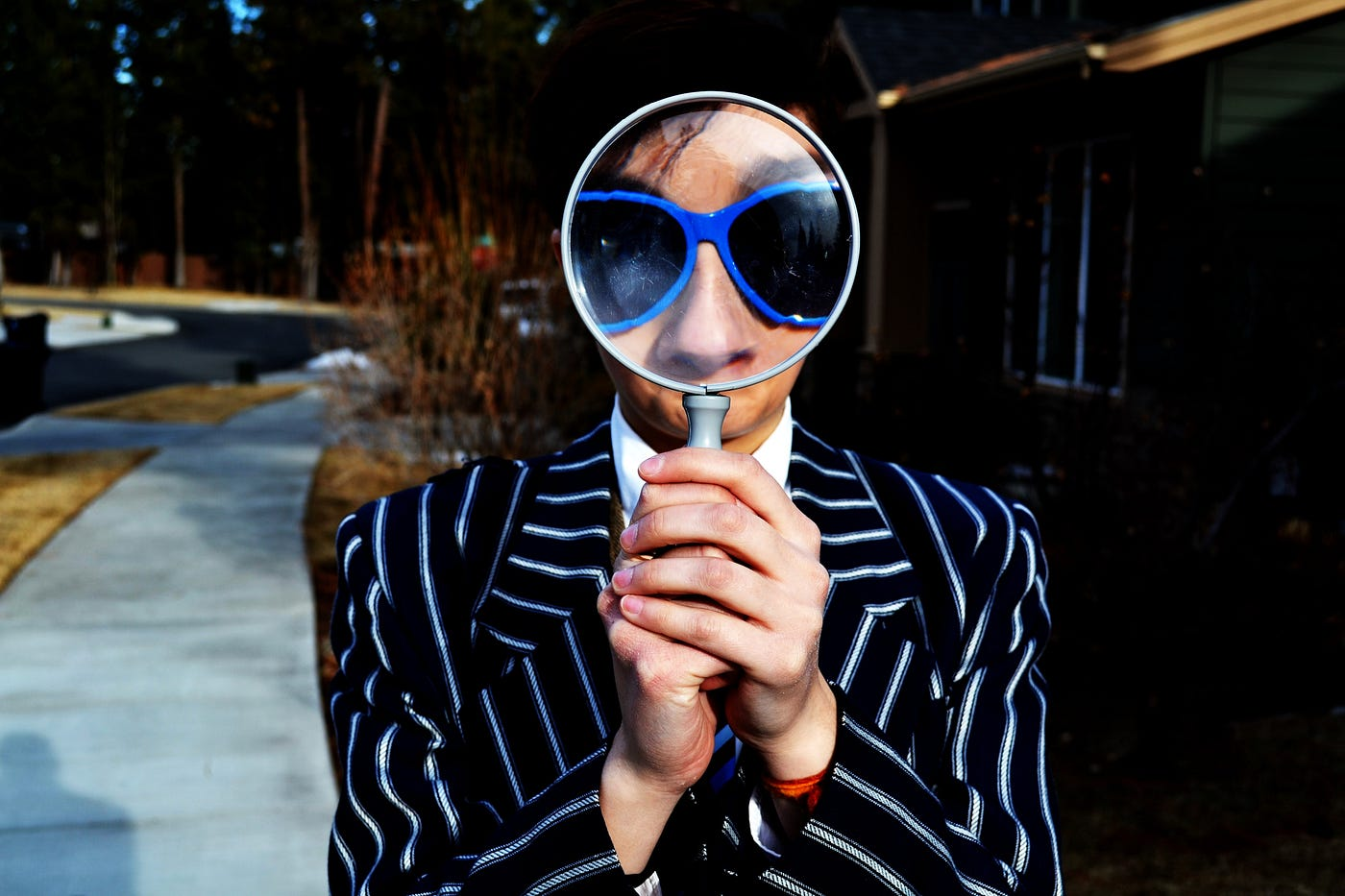Photo by Marten Newhall on Unsplash

This blog will provide an exhaustive study of YOLOv3 (You only look once), which is one of the most popular deep learning models extensively used for object detection, semantic segmentation, and image classification. In this blog, I’ll explain the architecture of YOLOv3 model, with its different layers, and see some results for object detection that I got while running the inference program on some test images using the model.

So keep reading the blog to find out more about YOLOv3.

## What is YOLO?

• “You Only Look Once” or YOLO is a family of deep learning models designed for fast object Detection.
• There are three main variations of YOLO, they are YOLOv1, YOLOv2, and YOLOv3.
• The first version proposed the general architecture, where the second version refined the design and made use of predefined anchor boxes to improve the bounding box proposal, and version three further refined the model architecture and training process.
• It is based on the idea that :

`" A single neural network predicts bounding boxes and class probabilities directly from full images in one evaluation. Since the whole detection pipeline is a single network, it can be optimized end-to-end directly on detection performance. "`

## 1. Network Architecture Diagram of YOLOv3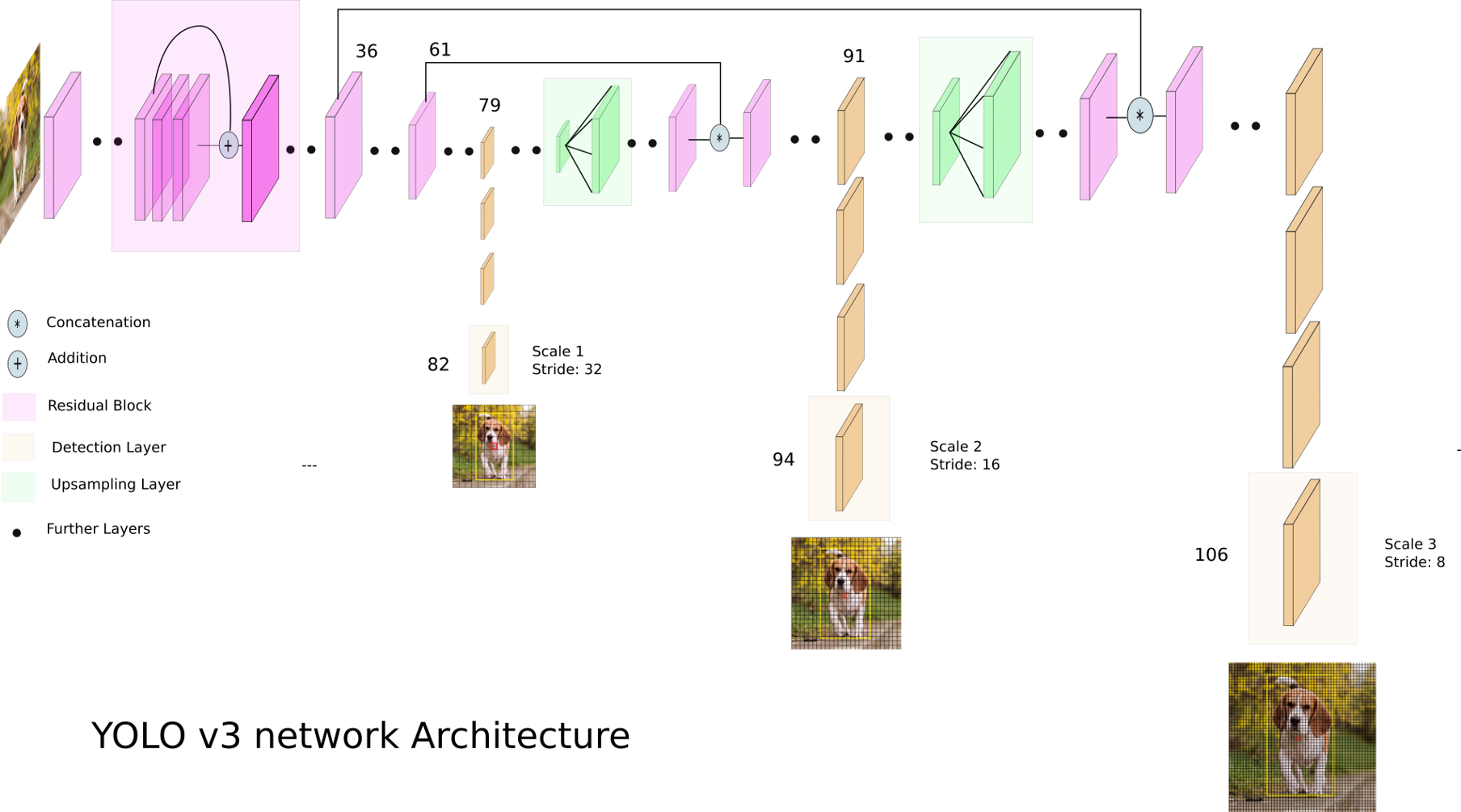## 2. Description of Architecture

#### Steps for object Detection using YOLO v3:

• The inputs is a batch of images of shape (m, 416, 416, 3).
• YOLO v3 passes this image to a convolutional neural network (CNN).
• The last two dimensions of the above output are flattened to get an output volume of (19, 19, 425):
• Here, each cell of a 19 x 19 grid returns 425 numbers.
• 425 = 5 * 85, where 5 is the number of anchor boxes per grid.
• 85 = 5 + 80, where 5 is (pc, bx, by, bh, bw) and 80 is the number of classes we want to detect.
• The output is a list of bounding boxes along with the recognized classes. Each bounding box is represented by 6 numbers (pc, bx, by, bh, bw, c). If we expand c into an 80-dimensional vector, each bounding box is represented by 85 numbers.
• Finally, we do the IoU (Intersection over Union) and Non-Max Suppression to avoid selecting overlapping boxes.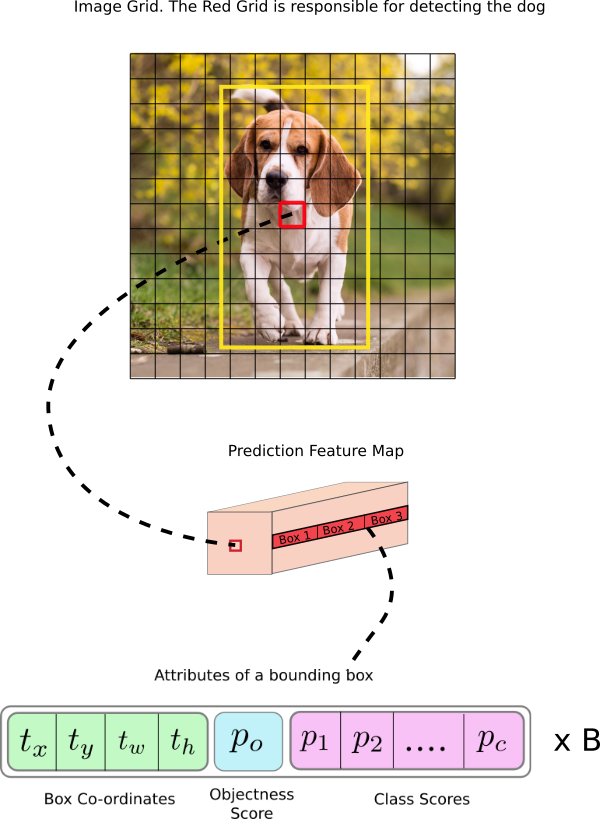#### Regarding the architecture:

• YOLO v3 uses a variant of Darknet, which originally has 53 layer network trained on Imagenet.
• For the task of detection, 53 more layers are stacked onto it, giving us a 106 layer fully convolutional underlying architecture for YOLO v3.
• In YOLO v3, the detection is done by applying 1 x 1 detection kernels on feature maps of three different sizes at three different places in the network.
• The shape of detection kernel is 1 x 1 x (B x (5 + C)). Here B is the number of bounding boxes a cell on the feature map can predict, ‘5’ is for the 4 bounding box attributes and one object confidence and C is the no. of classes.
• YOLO v3 uses binary cross-entropy for calculating the classification loss for each label while object confidence and class predictions are predicted through logistic regression.

#### Hyper-parameters used

• class_threshold - Defines probability threshold for the predicted object.
• Non-Max suppression Threshold - It helps overcome the problem of detecting an object multiple times in an image. It does this by taking boxes with maximum probability and suppressing the close-by boxes with non-max probabilities (less than the predefined threshold).
• input_height & input_shape - Image size to input.

### CNN architecture of Darknet-53

• Darknet-53 is used as a feature extractor.
• Darknet-53 mainly composed of 3 x 3 and 1 x 1 filters with skip connections like the residual network in ResNet.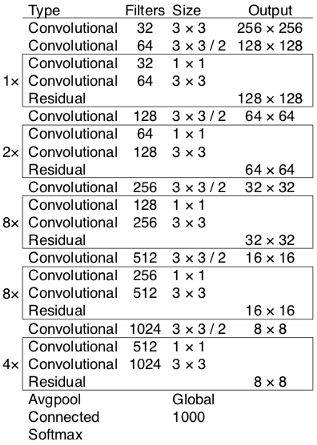### 2. Architecture diagram of YOLOv3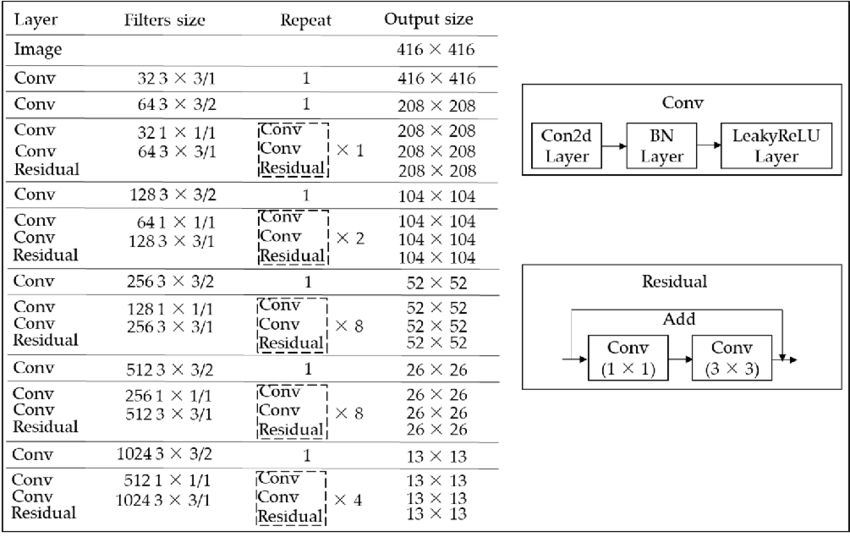### 3. Layers Details

• YOLO makes use of only convolutional layers, making it a fully convolutional network (FCN)
• In YOLOv3 a deeper architecture of feature extractor called Darknet-53 is used.

### Convolution layers in YOLOv3

• It contains 53 convolutional layers which have been, each followed by batch normalization layer and Leaky ReLU activation.
• Convolution layer is used to convolve multiple filters on the images and produces multiple feature maps
• No form of pooling is used and a convolutional layer with stride 2 is used to downsample the feature maps.
• It helps in preventing loss of low-level features often attributed to pooling.

### 4. Different Layers inside YOLO

• Code for Layer 1 to 53 in Tensorflow : Consider res_block() method for below code
``````def res_block(inputs, filters):
shortcut = inputs
net = conv2d(inputs, filters * 1, 1)
net = conv2d(net, filters * 2, 3)
net = net + shortcut
return net
``````

First two conv2d layers with 32 and 64 filters

``````net = conv2d(inputs, 32,  3, strides=1)
net = conv2d(net, 64,  3, strides=2)
``````

res_block * 1

``````    net = res_block(net, 32)
# Convolutional block with 128 filters
net = conv2d(net, 128, 3, strides=2)
``````

res_block * 2

``````for i in range(2):
net = res_block(net, 64)
# Convolutional layer with 256 filters
net = conv2d(net, 256, 3, strides=2)
``````

res_block * 8

``````for i in range(8):
net = res_block(net, 128)
# Convolutional layer with 512 filters
route_1 = net
net = conv2d(net, 512, 3, strides=2)
``````

res_block * 8

``````for i in range(8):
net = res_block(net, 256)
# Convolutional layer with 1024 filters
route_2 = net
net = conv2d(net, 1024, 3, strides=2)
``````

res_block * 4

``````for i in range(4):
net = res_block(net, 512)
route_3 = net
``````

### 5. Input Details for Model Inference

#### i. Input Preprocessing:

The images need to be resized to 416 x 416 pixels before feeding it into our model or the dimensions can also be specified while running the python file

#### ii. Input Dimensions:

The model expects inputs to be color images with the square shape of 416 x 416 pixels or it can also be specified by the user.

### 6. Details on Model Inference program and output

#### 1. The output of the model

• The output is a list of bounding boxes along with the recognized classes.
• Each bounding box is represented by 6 numbers (pc, bx, by, bh, bw, c). Here c is an 80-dimensional vector equivalent to the number of classes we want to predict, each bounding box is represented by 85 numbers.

#### 2. Output of Post-processing

• The Inference program produces a list of numpy arrays, the shape of which is displayed as the output. These arrays predict both the bounding boxes and class labels but are encoded.
• Further we will take each of the numpy arrays and decode the candidate bounding boxes and class predictions. If there are any bounding boxes that don’t confidently describe an object (having class probabilities less than the threshold, 0.3) we’ll ignore them.
• Here a maximum of 200 bounding boxes can be considered in an image.
• I have used the correct_yolo_boxes() function to perform translation of bounding box coordinates so that we can plot the original image and draw the bounding boxes.
• Again to remove those candidate bounding boxes that may be referring to the same object, we define the amount of overlap as non-max suppression threshold = 0.45.
• Also there is a need to rescale the coordinates of the bounding boxes to the original image along with displaying the label and scores on top of each of them.
• All these Post-processing steps need to be performed before we get the bounding boxes along with the recognized classes in our output image.

#### 3. Output of inference program for the model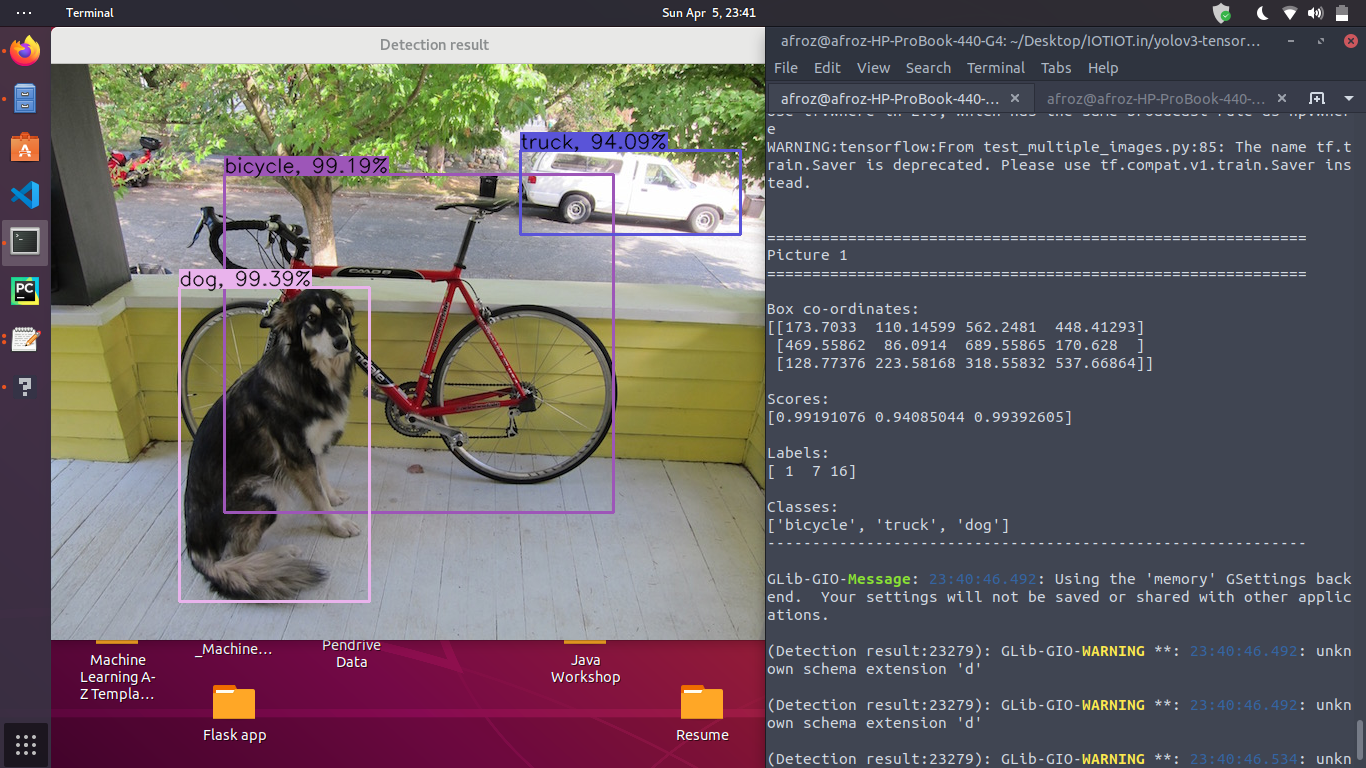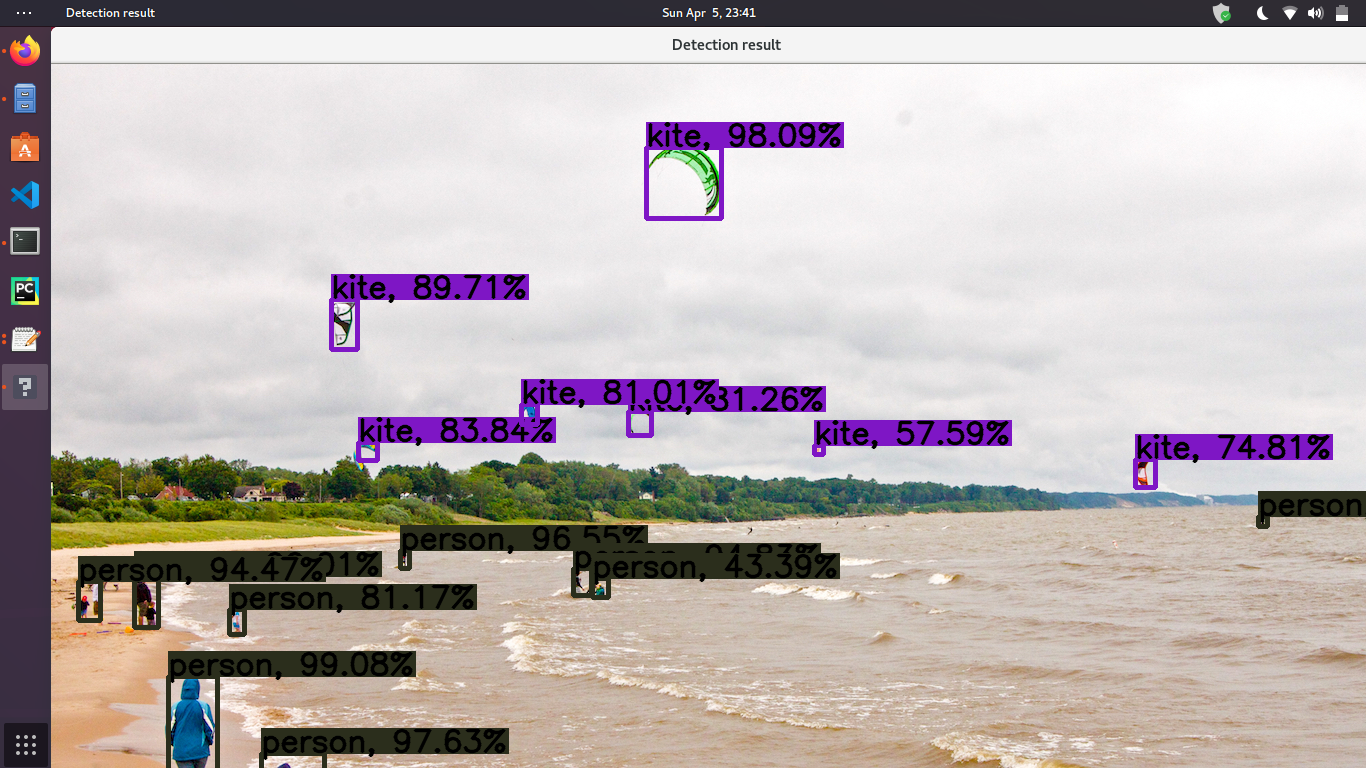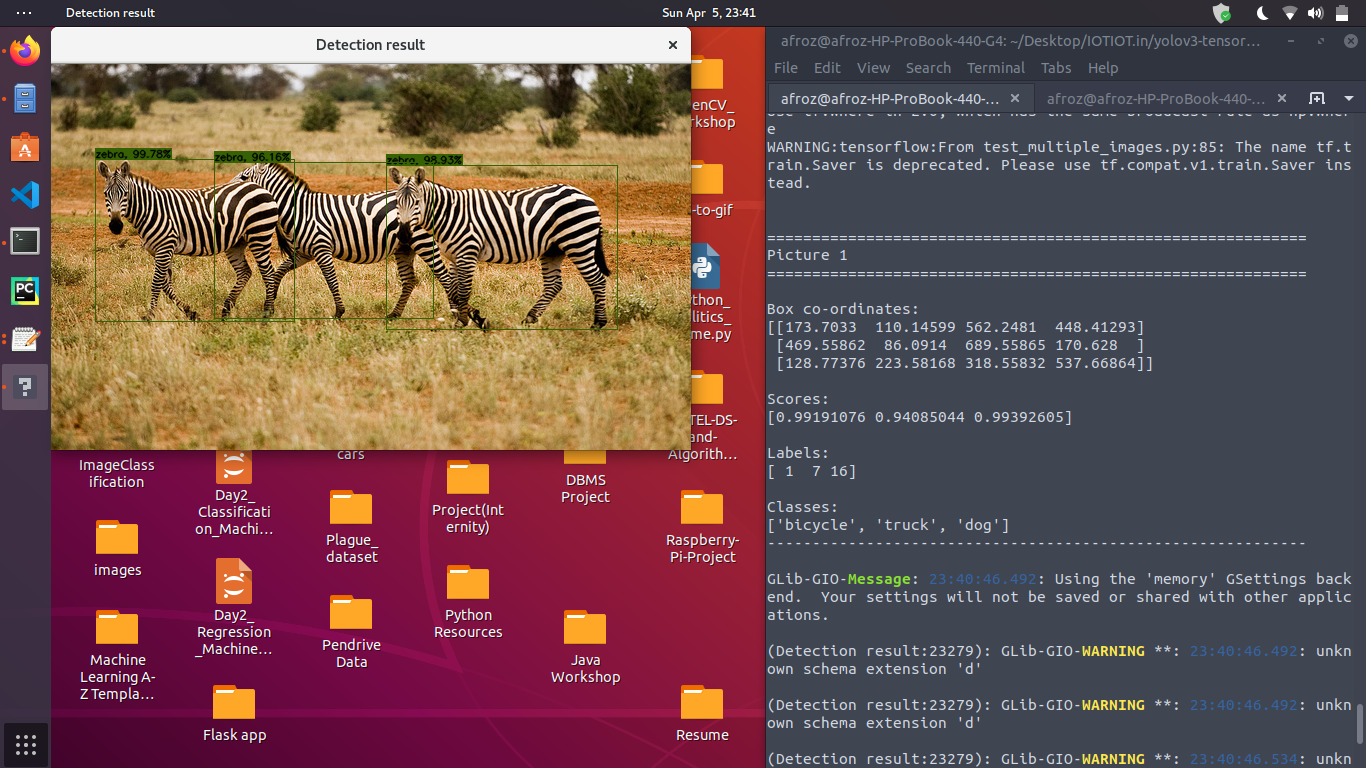### 7. Speed and Accuracy of YOLOv3

• Model Speed (in FPS) - On a Pascal Titan X it processes images at 30 FPS.
• Model Accuracy (On Testing dataset) - It has a mAP (Mean Average Precision) of 87.54% on VOC test set.

### 8. Run the model

Find link to my Dockerfile : afrozchakure/yolov3-tensorflow

To run the model using docker (docker should be preinstalled) on your machine use the below commands in the terminal :)

``````xhost +

sudo docker run --rm -ti --net=host --ipc=host -e DISPLAY=\$DISPLAY -v /tmp/.X11-unix:/tmp/.X11-unix --env="QT_X11_NO_MITSHM=1" afrozchakure/yolov3-tensorflow
``````

### 9. Learn more about Working Principles of YOLOv3 from below resources

Well done if you came this far. Cheers 😇💖

Tags:

Categories:

Updated: